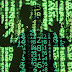This Module will hide files inside images ( currenlty PNG ) and export the modified image to disk
The maximum size of file which can be hidden inside an image depends on the dimension of the image.
``max_file_size = ( height_of_image * width_of_image * 6 / 8 ) bytes``
'100k words.txt' is hidden in 'original_image.png' named as 'image_with_100k words.png'. Go and checkout if you can spot the difference between these two images. You can use this Module to extract '100k words.txt' from the 'image_with_100k words.png'

Example
Original Image

Modified Image

This image has 100k words hidden inside it!

How it works?

It is based on a simple principle that if we change the LSB ( Least Significant Bits ) of every Pixel, then the change will not be significant and will not be noticed by eyes.
So, this Module takes 2 bits of data from the file to be hidden and replaces the last 2 bits of one pixel with those 2 bits, and then move to next pixel. The maximum change in pixel can be 4 units, and the range of values in a PNG Image if (0,255), so this change will not be significant.
In a PNG Image, each pixel has 3 channels Red, Green and Blue. ( Some PNG Images have 1 or 4 channels, but this Program will convert them to 3 channels) A typical pixel in a PNG Image looks like :
``a_pixel = (17,32,11)     # (RED, GREEN, BLUE)``
So, we can save 3 times 2 bits in a Pixel, that means 6 bits per pixel. That leads us to the upper limit of the file size, that can be hidden in an image:
``max_file_size = ( height_of_image * width_of_image * 6 / 8 ) bytes``
Let's understand by taking an example. The data to be hidden is :
``binary_data = 0b100111``
Let's take the first two bits and replace them with the RED Channel of our 'a_pixel'.
``````a_pixel = (0b10001, 0b100000, 0b1011)   # binary representation of a_pixel values
# Let's change a_pixel's RED Channel
# 0b10001 -> 0b10010  ( First 2 bits are 10 )
a_pixel = (0b10010, 0b100000, 0b1011)  # modified pixel``````
Let's again take 2 bits from binary_data and replace the last 2 bits of GREEN Channel with them.
``````a_pixel = (0b10010, 0b100000, 0b1011)
# Let's change a_pixel's GREEN Channel
# 0b100000 -> 0b100001  ( The 2 bits are 01 )
a_pixel = (0b10010, 0b100001, 0b1011)  # modified pixel``````
Let's do this one more time with BLUE Channel and we will be done.
``````a_pixel = (0b10010, 0b100001, 0b1011)
# Let's change a_pixel's BLUE Channel
# 0b1011 -> 0b1011  ( The 2 bits are 11 ) ; Notice that the value wasn't changed this time
a_pixel = (0b10010, 0b100001, 0b1011)  # pixel wasn't modified this time``````
So, we have hidden our 6 bit message in a pixel, let's see the changes in the pixel
``````a_pixel             = (0b10001, 0b100000, 0b1011) = (17, 32, 11)
a_pixel_with_data   = (0b10010, 0b100001, 0b1011) = (18, 33, 11)``````
As we can see that the change is not even noticeable at the pixel level. The Module do this repeatedly until all our data is saved to the image.

NOTE : The noise in the photo is increased and if we use any photo editing software and compare it with the original image, then this image will have much more noise than the original image.
Solution for this problem : Never ever upload/share the original image to internet

Todos
• Add support for JPEG Images
Steganographer - Hide Files Or Data In Image FilesReviewed by Zion3R on 5:30 PM Rating: 5